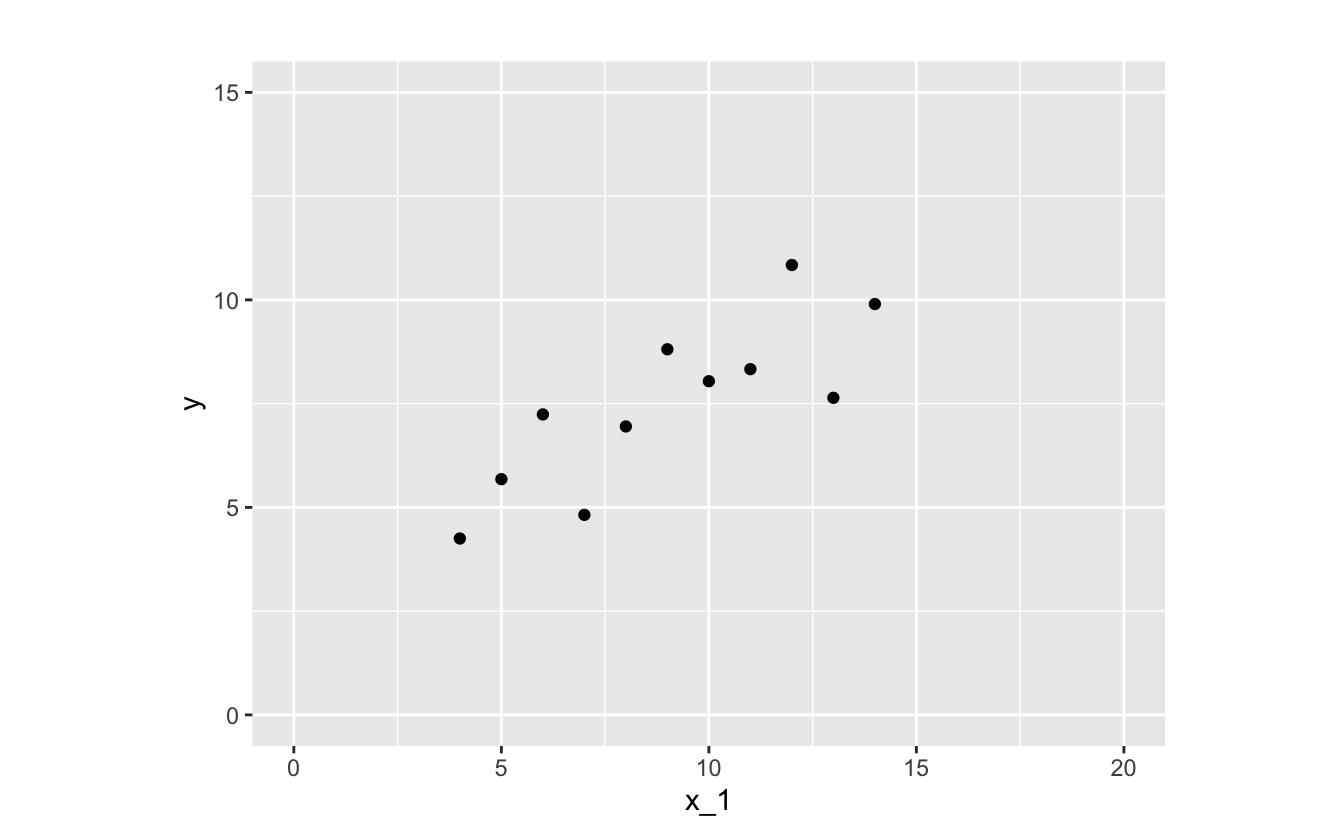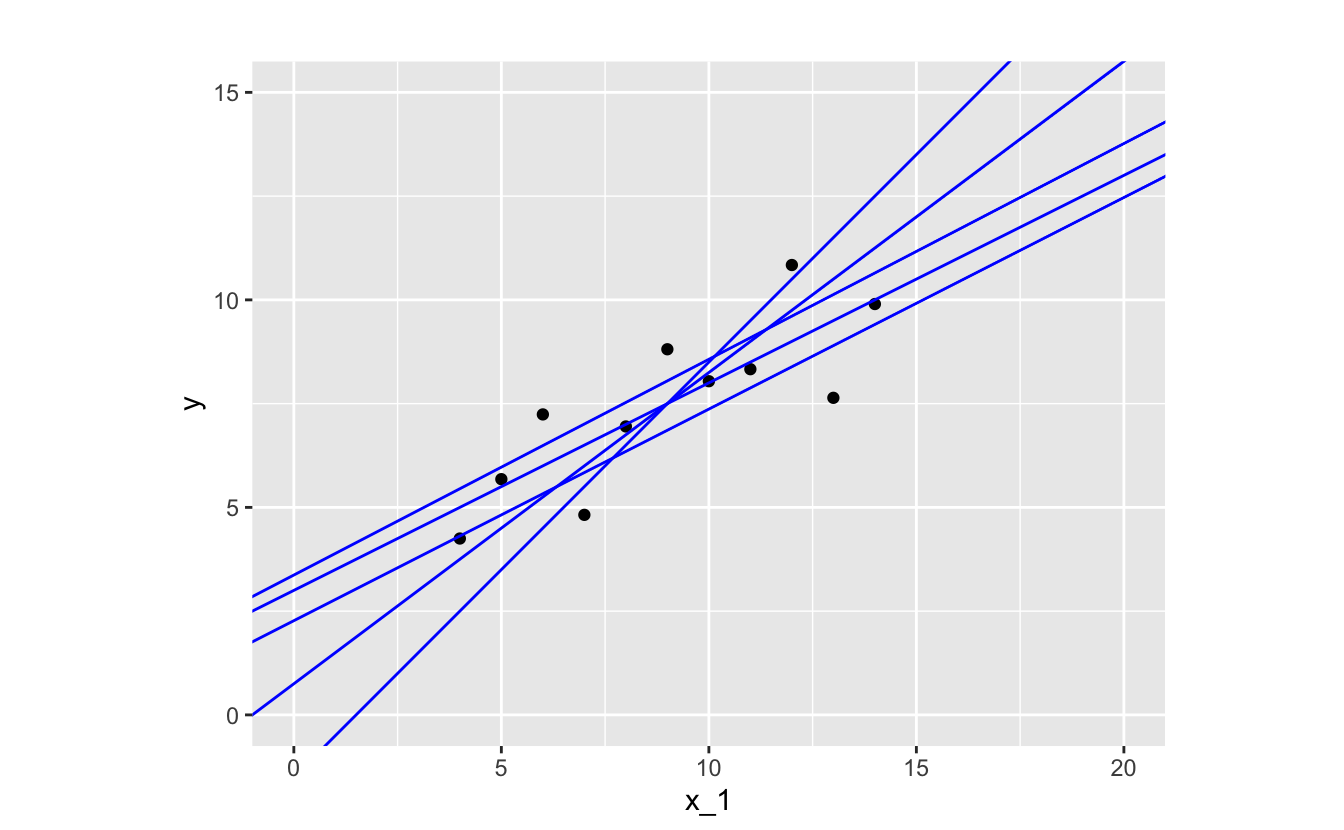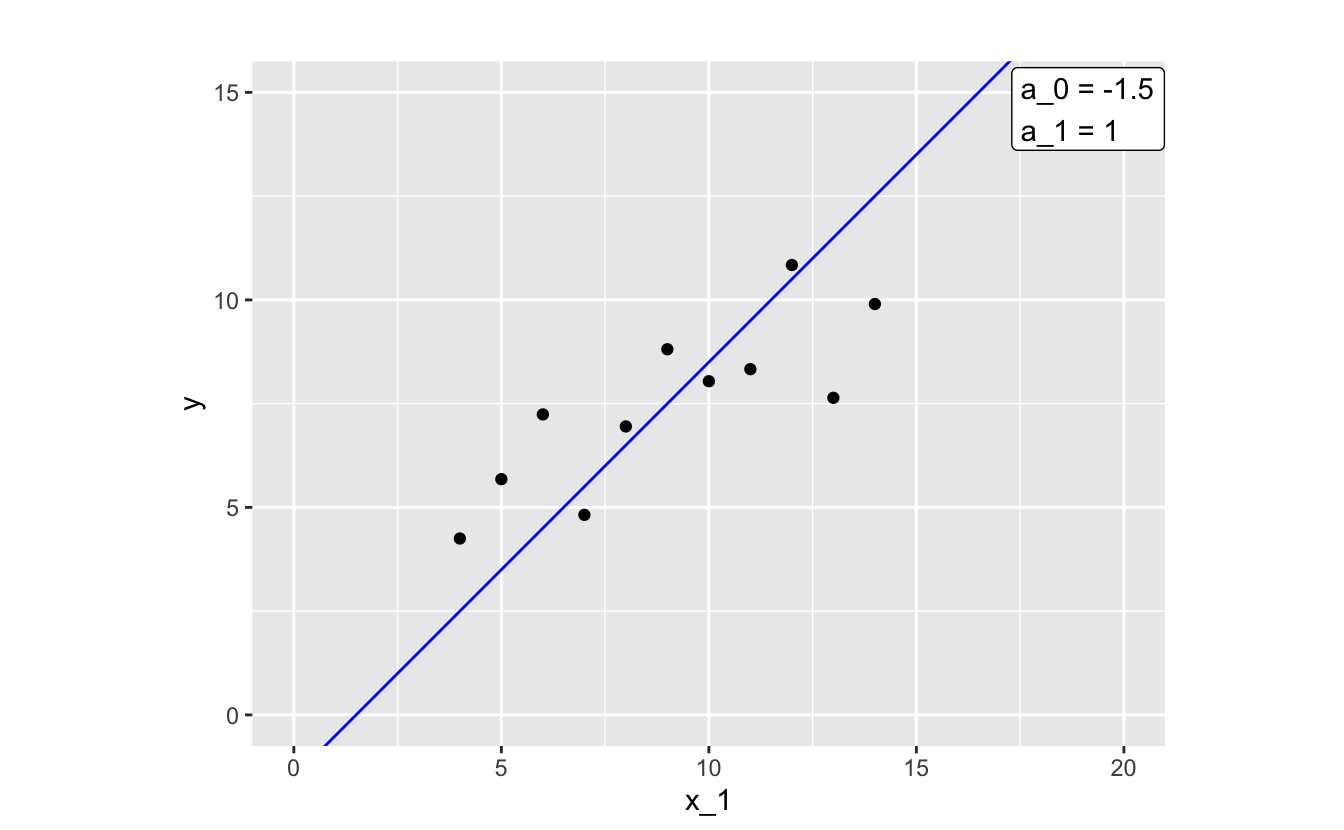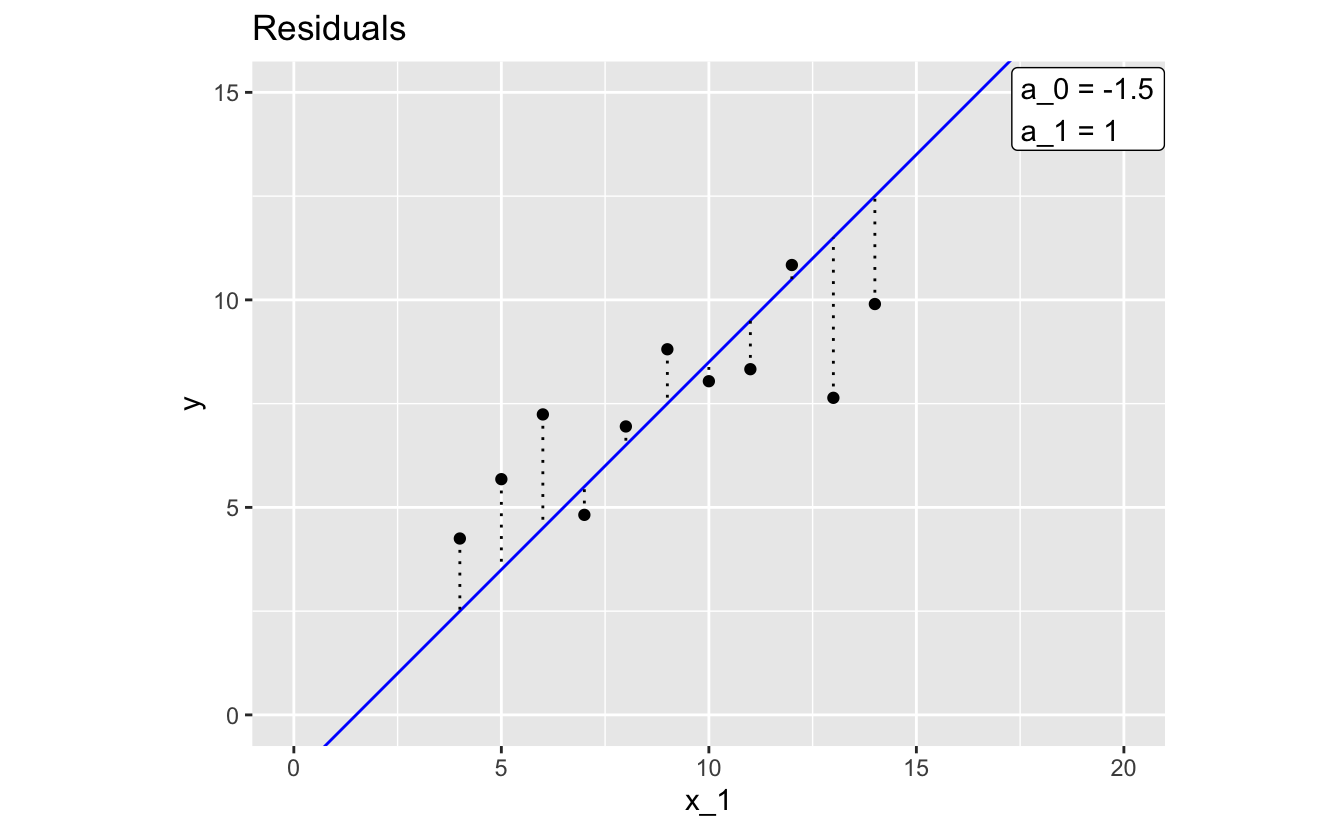# 1 Model basics

## 1.1 What is a model?

The world is complicated and messy, and there are endless details to even simple phenomena. To understand and navigate the world, we construct and use models.

For example, think about traffic. You probably have a simple mental model that says traffic will be worse at rush hour than at two in the morning. You can use this model to describe how traffic varies throughout the day, but you can also use it to predict the level of traffic at a given hour.

Your mental model of traffic, like any model, is an approximation. It tries to capture relevant information while ignoring noise and less important details. Your traffic mental model will never fully explain traffic, and so you’ll never be a perfect predictor of how many cars will be on the road at any given time. However, if it’s a good model, it will be useful.

The type of models we’ll discuss in this book are similar to your mental model of traffic: they are approximations; you can use them to both describe and predict the world; and, while they will never be completely accurate, they can be useful.

## 1.2 Supervised learning

You can divide up the world of models into two categories: supervised and unsupervised. In the following, we’ll discuss supervised learning, but it’s useful to understand the difference between the two.

Supervised models are functions. They approximate the relationship of one variable to others. The variable you want to explain (e.g., traffic) is called the response and the variables (e.g, time of day) you use to explain the response are called predictors.

Unsupervised models don’t have a response variable. Instead of trying to understand a relationship between predictors and a response, unsupervised models try to find patterns in data.

When building a supervised model, your job as the modeler is to find a function that approximates your data. Functions map from one or more variables (e.g., time) to another (e.g., amount of traffic). You can use your approximation function to understand and describe your data, as well as make predictions about your response variable.

There are an infinite number of types of functions, so how do you know where to look? The first step is to explore your data and determine with function family (or families) would best approximate your data. Function families are sets of functions with the same functional form. In the next section, we’ll talk more about the linear function family. Then, in Chapter 3, we’ll discuss how to use EDA to choose a function family to approximate your data.

## 1.3 Fitting a model

Anscombe’s quartet is a set of four small data sets. In this section, we’ll use a slightly modified version of the first data set in the quartet.

x_1 y
10 8.04
8 6.95
13 7.64
9 8.81
11 8.33
14 9.90
6 7.24
4 4.25
12 10.84
7 4.82
5 5.68

### 1.3.1 Decide on a function family

Recall that we said the first step to fitting a model is to determine which function family best approximates your data. In modeling, some of the most common and useful function families are linear. There are an infinite number of linear function families and, later, we’ll talk about how to decide exactly which family to use. For now, we’ll introduce the family of linear functions of a single variable. Functions in this family take the following form:

`y = a_0 + a_1 * x_1`

`x_1` is your input variable, the variable that you supply to the function in hopes of approximating some other variable. In our traffic example, `x_1` is the time of day.

`a_0` and `a_1` are the parameters. These two numbers define the line. The only difference between functions in the family of linear functions are their values of `a_0` and `a_1`.

To visualize this, here’s a plot of many different lines, each of which has a different combination of `a_0` and `a_1`.`a_0` defines the y-intercept, the y-value your function will produce when `x_1` is 0. `a_1` is the slope, which tells you how much `y` increases for every one unit increase in `x_1`. These two parameters define a linear function, and so to fit a linear model, you just have to determine which `a_0` and `a_1` best approximates your data.

As you’ll learn in Chapter 3, visualization is crucial to determining functional form. Let’s visualize the relationship between `x_1` (the predictor) and `y` (the response).The relationship between `x_1` and `y` looks linear, so a function in the linear family will likely make a good approximation.

### 1.3.2 Decide on an error metric

Now, we need a way of determining which linear function best approximates the relationship between `x_1` and `y`. There are many different functions we could use.How do we decide which one is best? Let’s just pick one of the lines and take a closer look.By glancing at the plot, you can tell that this function isn’t doing the best job of approximating our data. Most of the points where `x_1 < 9` fall above our line, and most of the points where `x_1 > 9` fall below the line.

To compare our chosen line to other possibilities, we need a way of quantifying how well the model approximates our data. A common way to assess model fit involves calculating the distances between the line and each of the data points.These distances are called residuals (or errors). Each residual represents the difference between the `y` value that the model predicts for a given `x_1` and the actual `y` associated with that `x_1`. The larger a residual, the worse your model approximates `y` at that value of `x_1`.

We need to turn all those residuals into a single error metric. One common option is to find the root mean-square error (RMSE). To calculate the RMSE, square each residual, find the mean, and then take the square root of that mean:

``sqrt(mean(residuals^2))``

The RMSE of our chosen model is 1.93. We could calculate the RMSE of each model we originally plotted and pick the one with lowest value. However, because there are an infinite number of possible models, that method won’t necessarily result in finding the model that minimizes RMSE. Instead, we’ll hand off the work to an algorithm (implemented in an R function) that will return the values of `a_0` and `a_1` that minimize RMSE for our data.

To determine which `a_0` and `a_1` minimize RMSE, we’ll use the function `lm()`. `lm()` needs two arguments: a function family and your data. Then, it finds the parameters of the function within your specified family that minimize RMSE for your data. In later chapters, you’ll learn how to actually carry this out in R. For now, we’ll just show you the results.

Here’s the model that `lm()` came up with:

`y = 3 + 0.5 * x_1`

And here’s the model plotted against our data:The RMSE of the model found with `lm()` is 1.11. You can also tell from the plot that this fitted model does a much better job of approximating our data than our previous attempt.

Again, `a_0` is the intercept, so we know that our model predicts that `y` will be 3 when `x_1 = 0`. `a_1` is the slope, which means that our model predicts a 0.5 increase in `y` each time `x_1` increases by 1.

Now, we’ll talk more about error metric choice.

## 1.4 Error metric choice

One downside of models that minimize RMSE is that they’re very sensitive to outliers. The following plot shows our original data plus an outlier.If we keep using RMSE as our error metric, this single point changes the model significantly.The outlier pulls the slope down and the intercept up, and the model no longer approximates the rest of the data well. This plot demonstrates why it’s important to visualize your model’s predictions. If you only looked at the model’s RMSE, you might think it was doing a good job of approximating your data.

We want to approximate the linear trend present in the rest of the data, so we need a way to build a better model. There are two options:

• Use a different error metric.
• Exclude the outlier from the data.

First, we’ll try a different error metric. Because RMSE squares each residual, a single large residual can disproportionately influence the model. Other error metrics, such as mean absolute error (MAE), are less sensitive to outliers. Instead of squaring each residual, MAE finds their absolute values:

``mean(abs(residuals))``

We’ll fit another model on the outlier data, using MAE as our error metric instead of RMSE.The MAE model isn’t as disproportionately affected by our outlier as the RMSE model. `lm()` does not allow you to use MAE as your error metric, but `MASS::rlm()` (the “r” is for “robust”) offers a range of error metrics that, like MAE, are less sensitive to outliers than RMSE.

The MAE model is similar to the model that we fit on the data without the outlier, which brings us to the second approach: remove the outlier. We’ll talk more about this in the next chapter.

## 1.5 Summary

In this chapter, we went over the basic process of fitting a model. Here are the steps again:

• Explore your data and choose an appropriate function family.
• Choose an error metric.
• Use an R function to find the specific function in the function family that minimizes the error metric.

Before you do any of these three steps, however, you first have to understand your data and check for any problems. The simple data used in this chapter doesn’t measure anything real, but you’ll typically be fitting models to complex, messy data sets. In the next chapter, we’ll discuss how to use exploratory data analysis to understand and prepare your data before starting the modeling process.